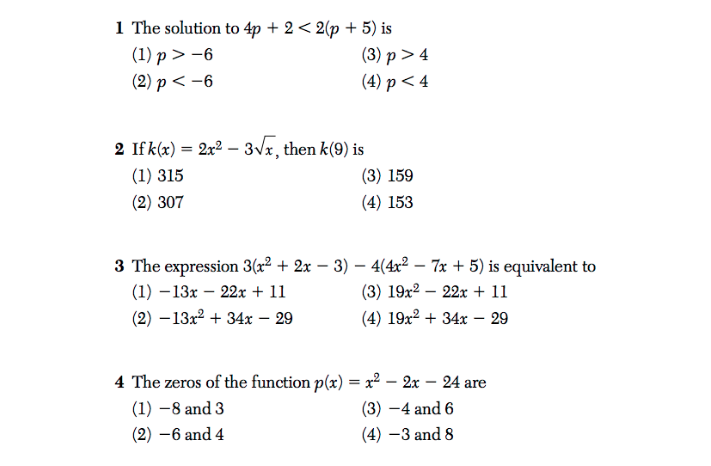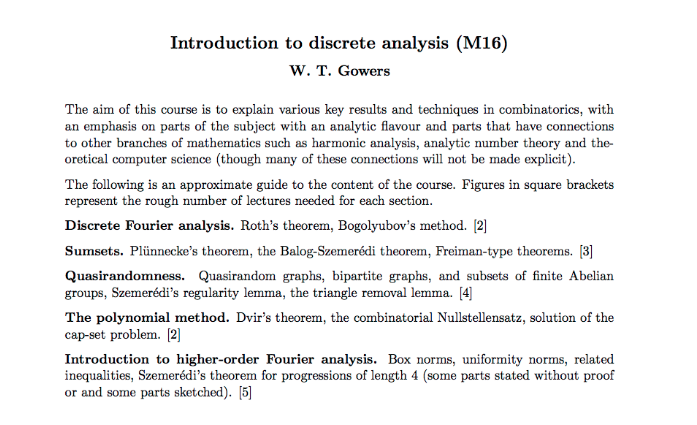Education ∪ Math ∪ Technology

This post is part of the Virtual Conference on Mathematical Flavors, and is part of a group thinking about different cultures within mathematics, and how those relate to teaching. Our group draws its initial inspiration from writing by mathematicians that describe different camps and cultures — from problem solvers and theorists, musicians and artists, explorers, alchemists and wrestlers, to “makers of patterns.” Are each of these cultures represented in the math curriculum? Do different teachers emphasize different aspects of mathematics? Are all of these ways of thinking about math useful when thinking about teaching, or are some of them harmful? These are the sorts of questions our group is asking.

***

There are many cultures of mathematics, a question is, which one dominates k-12 education and why?

The majority of those two cultures are likely aligned to one of these two possibly overlapping goals of teaching mathematics.

1. To enable students to be able to solve a specific set of mathematical problems.
2. To teach students a body of mathematics.

If you look at what is assessed in mathematics, the majority of assessment focuses on students being able to solve specific mathematical problems. Here are some examples of problems that New York State feels are so important for students to be able to solve that they gave these questions to the tens of thousands of student who sat for the June 2018 Algebra I Regents examination.I do not know if it is possible to assess students on their understanding of mathematical principles without giving them some type of problem to solve, but I have never seen an assessment that did not involve solving mathematical problems, so it is either systemic in the way mathematics is assessed or it is impossible to assess mathematical principles apart from problems they can applied to solve.

I have occasionally seen teaching that focused on students building understanding of mathematical principles rather than attempting to solve specific problems, but even in this case the basis of the activity could be considered a mathematical puzzle or problem. However, almost every time I have observed a class the focus is on students being able to solve a fairly specific set of problems with little to no opportunity to generalize from the experience.

According to Tim Gowers, in his essay Two Cultures of Mathematics, these two same cultures apply in the world of mathematicians as well, except the culture that dominates is reversed; most mathematicians focus on learning more mathematical principles and eschew pure problem solving as a goal.

As Sir Michael Atiyah (Atiyah, 1984) puts it:

MINIO: How do you select a problem to study?

ATIYAH: I think that presupposes an answer. I don’t think that’s the way I work at all. Some people may sit back and say, “I want to solve this problem” and they sit down and say, “How do I solve this problem?” I don’t. I just move around in the mathematical waters, thinking about things, being curious, interested, talking to people, stirring up ideas; things emerge and I follow them up. Or I see something which connects up with something else I know about, and I try to put them together and things develop. I have practically never started off with any idea of what I’m going to be doing or where it’s going to go. I’m interested in mathematics; I talk, I learn, I discuss and then interesting questions simply emerge. I have never started off with a particular goal, except the goal of understanding mathematics.

So if mathematicians value mathematics as a set of organizing ideas and mostly prefer to study the organizing ideas rather than the problems they can be used to solve, then why does almost all of k-12 mathematics focus primarily on problems to be solved and much less so on general mathematical principles?

Maybe the problem lies with the standards that kindergarten to grade 12 teachers are expected to focus on? Let’s take a look at the Common Core clusters each of the questions listed above is supposed to correspond, which are A-REI.B, F-IF.A, A-APR.A, and A-SSE.B respectively.

The text of those clusters is as follows:

• A-REI.B: Solve equations and inequalities in one variable.
• F-IF.A: Understand the concept of a function and use function notation.
• A-APR.A: Perform arithmetic operations on polynomials.
• A-SSE.B: Write expressions in equivalent forms to solve problems.

It certainly seems like 3 out of 4 of those are focused on students being able to use what they know to solve specific kinds of problems with verbs like solve, perform, and write. So the fault could lie with how standards are written for courses.

Let’s contrast these standards with the objectives from one of the courses Tim Gowers teaches to see how they compare:One obvious difference between the standards and Gowers’ syllabus is the inclusion of verbs in the standards, which besides being the intended foci of a high school algebra course are also intended to be a map for people writing assessments, and you cannot write an assessment without specifying what students will do in order to demonstrate understanding. Gowers’ syllabus is just a list of topics, but I note that I have no idea based on his syllabus what kinds of problems these topics would be useful for and maybe the absence of verbs is why.

There is a way of teaching that breaks this dichotomy between solving problems and learning mathematical principles and uses problems to teach mathematical principles but this style of teaching seems incredibly rare and is somewhat difficult to learn and somewhat difficult to do. The best tool I have found for learning this kind of teaching is through the use of instructional routines, particularly an instructional routine like Connecting Representations that focuses on students making connections between two different representations of the same mathematica principle.

I’m not really sure why this difference between the work of mathematicians and the goals of k-12 mathematics teachers exists. One possibility is that it’s a lot easier to see how mathematics can be applied to solving problems than it is to see the mathematical principles being used and very few k-12 mathematics teachers are as mathematicians.

References:

1. T. Gowers, The Two Cultures of Mathematics, Mathematics: Frontiers and Perspectives (2000)
2. F. Atiyah, An interview with Michael Atiyah, Math. Intelligencer 6 (1984), 9-19.